# What is Linked List Data Structure

## What is Linked List Data Structure?

1. What is a linked list?
2. What is a node?
3. Node structure
4. Create a Node
5. Access Node

##### What is Linked List Data Structure?

A linked list is a collection of nodes where each node contains data and a pointer to the next node.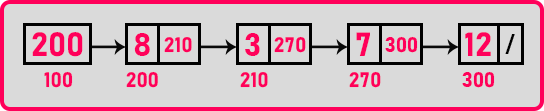If you see this 1st node, its address is ‘200’ and it is having data ‘8’ and address ‘210’. It is the address of the next node. The 2nd node is having the address ‘210’, data is ‘3’, and the address of the next node is ‘270’. Again the 3rd node is having the address ‘270’, data is ‘7’, and the address of the next node is ‘300’. And the last node is having the address ‘300’, data is ‘12’ and as there are no elements after this, we store null in the address of the next node.

‘First’ is a pointer that points on the 1st node. We call this pointer name ‘First’ or also we call it ‘Head’. The address of ‘Head’ or ‘First’ is ‘100’. Now, this link list that we have shown you is similar to the one already we have discussed in the previous article that the linked list was created inside a heap.

These addresses are just we have taken randomly. In real when you create some memory in the heap that may be in thousands. But for making it simple we have taken their addresses in hundreds. So, these are just random addresses.

Now one thing you can observe in an array all the addresses are contiguous (side by side). If one is ‘200’ then next will ‘202’ and so on. But in a linked list, if one address is ‘200’ then next is ‘210’, and next will ‘270’. So, all those nodes are not sided by side. Then how the continuity is maintained? It is maintained through links (pointer to next node) that are addressed. This is about a linked list and it is created in heap.

##### How to create a node?

We have to define two things’ data and a pointer. Data may be any type like int, float, double, or any other type. Then pointer is which type? The pointer is pointing to a node, so the pointer is of the node type. The node will be having a pointer of the node type. It’s a pointer of its own type.

##### Structure of the node

Now let us define the structure of the node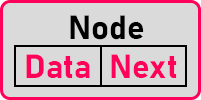Here we say pointer to next node as ‘next’. So, this is node structure. Node is having data and pointer to next node then in programming how we can define this a structure. So, for that, we can use C language as a structure

struct Node;

Data and Pointer to the next node are the members of this structure. Data can be of any type. You can pick anything you want. Usually, for a learning purpose, we use integers, so we will call it integer data. The Next pointer will be of type ‘Node’. Now you can see that structure is having data as well as a pointer of type structure node only.

struct Node{
int data;
struct Node *next;
};

So, it’s having a pointer of its own type so such structure is called a self-referential structure. Self-Referential Structures are used in the linked lists. For C language programming we use a structure and for C++ programming also we can use a structure as it also supports the structure.

##### What is the structure in C++?

It is the same as class. Then what is the difference between class and structure? In class everything by default is private and in structure everything by default is public. If you are writing a C++ program then there also, we can define a node with a structure or with a class also.

##### Now next thing what is the size of this structure?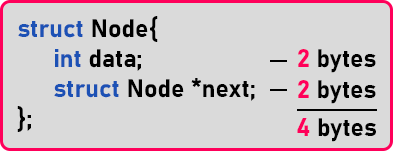Data is of ‘int’ type and we are assuming every time that integer takes 2 bytes. In any compiler, if the integer is taking ‘2 bytes’ then the pointer will also take ‘2 bytes’. So, the size of the structure is 4 bytes. It means this node is occupying ‘4’ bytes. Every node will consume ‘4’ byes in the linked list. Now next let us see how these nodes are created.

##### How to create Nodes?

For creating a node, first of all, we need a pointer because nodes have to be created inside the heap.

struct Node *p;

So, we have taken a pointer. We are calling the pointer ‘p’. When we declare any variable, the memory will be allocated inside the stack. Now we have to create a node in the heap. For that, we know the ‘malloc’ function will use here to allocate memory in the heap. So, we will write it as,

struct Node *p;
p = (struct Node*) malloc (sizeof(struct Node));

This ‘malloc’ function will return a ‘void’ type of pointer. So, we have to typecast it to the ‘Node’ type. This is very length code, this is how we do it in C language but in C++ it’s very simple,

p = new Node;

In C++, with the ‘new’ keyword, we can allocate memory in heap.

###### Example of node: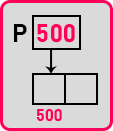So, we have seen how to create a node then how do access the members of a node?

##### How to access members of a node?

For writing data in a node,
p -> data = 20;
p -> next = 0;

The members are accessed using the ‘->’ symbol. So, this is all we can access the members of a structure. This is how we create a node and access the members of Node.

##### Important Statements related to Linked List Data Structure:

Let us learn a few more important statements that are useful when we are accessing the linked list. Let us take an example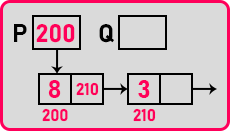Now let us see some syntax and understand their meaning.

Q = P;

What does it mean? It means whatever is there in ‘P’ has to be stored in ‘Q’ also. Here ‘Q’ will have the address ‘200’ and ‘Q’ will be pointing on the same node where ‘P’ is pointing.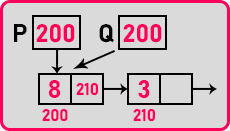‘Q’ assigned to ‘P’ means ‘Q’ is pointing on the same node where ‘P’ is pointing. Now let us look at the next syntax

Q = P->next;

What does it mean? Inside ‘Q’ we want to store ‘P’s next’. So, what is P’s next? P’s next is having the address of the next node that is ‘210’. This ’210’ will save in ‘Q’. Now, ‘Q’ is pointing to the P’s next node.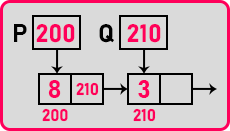Now one more statement:

P = P->next;

We have already seen that ‘Q’ points on the next node of ‘P’. So here what is the meaning of this? It means storing the value of P’s next in P itself. P’s next is ‘210’ so it will store in ‘P’. Now ‘P’ will point on,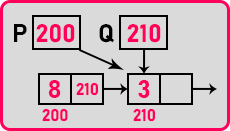The statement ‘P = P->next;’ has made ‘P’ move to the next node. This is a very useful statement in the linked list.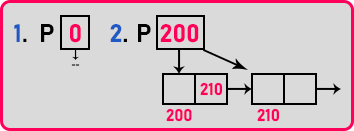Now we have two examples. In 1st example ‘P’ is not pointing anywhere it is null or the address is zero and in 2nd example, ‘P’ is pointing on some node in a linked list. How to make ‘P’ null? We can write ‘0’ as

struct Node *P = 0;

or else we can write,

struct Node *P = NULL;

Now let us see those two examples. If pointer ‘P’ is not pointing on any node then it will have a value ‘0’ that is null. If it is pointing on some node then it will have some valid address and that value will not be 0, it will non-zero and in C / C++ programming zero means False and any other non-zero value means True.

So, if pointer ‘P’ is not pointing on any node then it is zero, which means it is false and when pointing on some note it will have the non-zero value that means it is true. Now let us see the conditions to check if a pointer pointing on some node or not.

###### 1st condition:

If (P == null)

In which example this condition will be true? In 1st example, ‘P’ is equal to null. So, this means it will be true if pointer ‘P’ is not pointing to any node.

###### 2nd condition:

If (P == 0)

Again, this will be true in 1st example where ‘P’ is not pointing anywhere.

###### 3rd condition:

If (!P)

Here also 1st example will be true.

So, these are the three methods of checking if a pointer is not pointing to any node or not pointing anywhere. If the pointer is null then these conditions will be true. Let us write the inverse of the above 3 conditions as

If (P != null)

If (P != 0)

If (P)

The above 3 conditions will be true for 2nd example where ‘P’ is pointing on some node. These are a few important conditions. And we have one more condition. We want to know whether after node ‘P’ there are some more nodes or not. So let us see this.

If (P->next == null)

This will check if the node next has some other nodes or it is null. If there is no more node then the current node is the last node of the linked list. Another condition is

If (P->next != null)

It will be true if there is another node present after the current node. So, these are a few important statements and conditions that are commonly used while working with Linked lists.

In the next article, I am going to discuss How to Display a Linked List in C Language with Examples. Here, in this article, I try to explain What is Linked List Data Structure and I hope you enjoy this What is Linked List Data Structure article.